## Area

• The term 'area' is a quantity giving the size of a two-dimensional region or shape within a given enclosed border.

The formulae for the area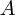of common shapes are:

 shape formula note square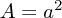where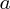is the length of one of the sides rectangle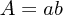whereis the base and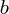is the height circle=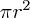where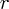is the radius triangle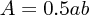whereis the base andis the height